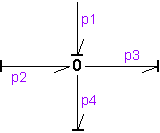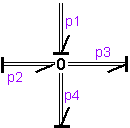﻿ 20-sim webhelp > Library > Bond Graph > ZeroJunction

# ZeroJunction

Bond Graph

## Use

Domains: Continuous. Size: [n,m]. Kind: Bond Graphs.

## Description

This junction represents a power continuous (no energy storage, dissipation or generation) connection of elements. The sum of the flows on all ports is zero and the efforts on all ports are equal. The constitutive equations are for example:p1.f + p2.f - p3.f - p4.f = 0;   p1.e = p2.e = p3.e = p4.e;   effort = p1.e;

A zero junction has only one initial port p defined. Because any number of bonds may be connected, successive connected bonds are named p1, p2, p3 etc.

The plus or minus signs of the flow equation depend on the direction of the bonds. A bond pointing towards the zero junction results in a plus sign and vice-versa. Only one port of a zero junction may determine the effort. In the example port p1 determines the effort.

## Multi Bonds

Bonds with a size larger than one (multi bonds) can also be connected to a zero junction. Al connected bonds, however, must have the same size.p1.f + p2.f - p3.f + p4.f = 0;   p1.e = p2.e = p3.e = p4.e;   effort = p1.e;

Naming conventions are equal to those of the single bond (size 1) junctions. Successive connected bonds are named p1, p2, p3 etc. To denote single elements of a multibond, matrix notation is used. E.g the effort of bond number 3 of a multibond p2 can be denoted as p2.e (columnvector notation) or p2.e[3,1] (matrix notation).

## Interface

Ports

Description

p[any]

Any number of ports may be connected.

## Causality

1_flow p

Only one port may have a flow out causality (as seen from the element).

## Outputs

effort

The output signal is equal to the effort of the ports.

## Limitations

All connected bonds must have the same size.